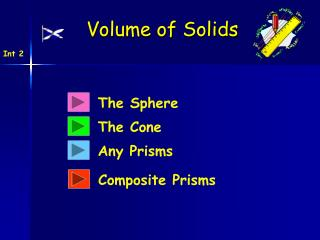DownloadDownload PresentationThe Sphere

The Sphere

Télécharger la présentationThe Sphere

- - - - - - - - - - - - - - - - - - - - - - - - - - - E N D - - - - - - - - - - - - - - - - - - - - - - - - - - -
Presentation Transcript

1. Volume of Solids Int 2 The Sphere The Cone Any Prisms Composite Prisms

2. Int 2 Starter 5 cm 3 cm 2.5 cm 3 cm 4 cm

3. Volume of Solids Int 2 Learning Intention Success Criteria • To know the volume formula for a sphere. • To understand the • sphere formula and use it • appropriately. • Work out volumes for spheres. • Answer to contain • appropriate units and working.

4. Volume of a Sphere Int 2 D = diameter r D Q. If the above sphere has radius 10cm. Calculate it’s volume.

5. Volume of Solids Int 2 Now try MIA Ex 1 Ch3 (page 20)

6. r D Int 2 Starter D = 6 cm

7. Volume of Solids Int 2 Learning Intention Success Criteria • To know the volume formula for a cone. • To understand the • cone formula and use it • appropriately. • Work out volumes for various cones. • Answer to contain • appropriate units and working.

8. Volume of a Cone Int 2 h r • If the above cone has radius 15cm and height • of 10 cm. Calculate it’s volume.

9. Volume of Solids Int 2 Now try MIA Ex 2 Ch3 (page 22)

10. Int 2 Starter 6 cm r = 3cm 5 cm 8 cm 6 cm

11. Volume of Solids Prisms Int 2 Learning Intention Success Criteria • To know the volume formula for any prism. • To understand the • prism formula for calculating volume. • Work out volumes for • various prisms. • Answer to contain • appropriate units and working.

12. Volume of Solids Int 2 Definition : A prism is a solid shape with uniform cross-section Hexagonal Prism Cylinder (circular Prism) Triangular Prism Pentagonal Prism Volume = Area of Cross section x length V = A x l

13. Volume of Solids Int 2 Definition : A prism is a solid shape with uniform cross-section Q. Find the volume the triangular prism. Triangular Prism Volume = Area x length V = 20 x 10 = 200 cm3 10cm 20cm2

14. Volume of Solids Int 2 Definition : A prism is a solid shape with uniform cross-section Q. Find the volume the hexagonal prism. 43.2cm2 Volume = Area x length 20cm Hexagonal Prism = 43.2 x 20 = 864 cm3

15. Volume of Solids Int 2 Now try MIA Ex 3,4 Ch3 (page 24/25)

16. h r Int 2 Starter D = 6 cm h = 10 cm

17. Volume of Solids Prisms Int 2 Learning Intention Success Criteria • To know what a composite volume is. • To calculate volumes for composite shapes using knowledge from previous sections. • Work out composite volumes using previous knowledge of basic prisms. • Answer to contain • appropriate units and working.

18. r h D r Volume of a Solid Int 2 Composite volume is simply volumes that are made up from basic volumes. Volume = Area x length

19. r 2m Volume of a Solid Int 2 Composite volumes are simply volumes that are made up from basic volumes. Q. Find the volume the composite shape. Volume = Cylinder + half a sphere h = 6m

20. Volume of a Solid Int 2 Q. This child’s toy is made from 2 identical cones. Calculate the total volume. Volume = 2 x cone r = 10cm h = 60cm